# Linear Graphs

• Location of a Point
• Coordinates

## Definition

• Linear graph: A line graph which is a whole unbroken line is called a linear graph.
• Cartesian system: The system used to describe the position of a point in a plane is called the Cartesian system.
• Origin: The point of intersection of x and y lines is called the origin.
• Abscissa: X-coordinate tells how many units to move right or left. It is also called the Abscissa.
• Ordinate: Y-coordinate tells how many units to move up or down. It is also called the Ordinate.
• Cartesian Coordinate: X-coordinate and y-coordinate taken together are called cartesian coordinates or coordinates of a point and denoted by (x, y).
• Ordered pair: The x-coordinate comes first, and after this y-coordinate comes. (x, y) is called an ordered pair.

## Notes

### Linear Graphs:

A line graph which is a whole unbroken line is called a linear graph.

#### 1. Location of a point:

• The system used to describe the position of a point in a plane is called the Cartesian system.

• In the Cartesian system, there are two perpendicular directed straight lines XX’ and YY’ which intersect at point 0, then line XX’ will be a horizontal line and YY’ will be a vertical line.

• The point of intersection of these lines is called origin and it is denoted by O. In other words, the point from which distances are marked is called an origin.

• The horizontal line XOX’ is called X-axis and the vertical line YOY’ is called Y-axis.

• Directions OX and OY are called the positive directions of the X-axis and Y-axis, respectively, and directions OX’ and OY’ are called the negative directions of the X-axis and Y-axis, respectively.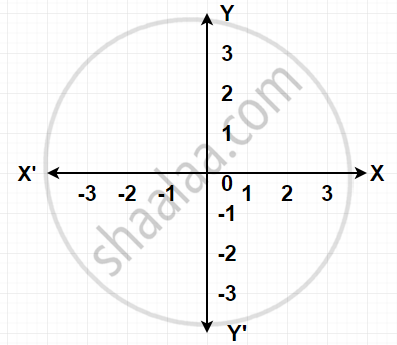#### 2. Coordinates:

• For fixing a point on the graph sheet we need, x-coordinate and y-coordinate.

• x-coordinate tells how many units to move right or left. It is also called the Abscissa.

• y-coordinate tells how many units to move up or down. It is also called the Ordinate.

• x-coordinate and y-coordinate taken together are called cartesian coordinates or coordinates of a point and denoted by (x, y). Here, the x-coordinate comes first, and after this y-coordinate comes. (x, y) is called an ordered pair.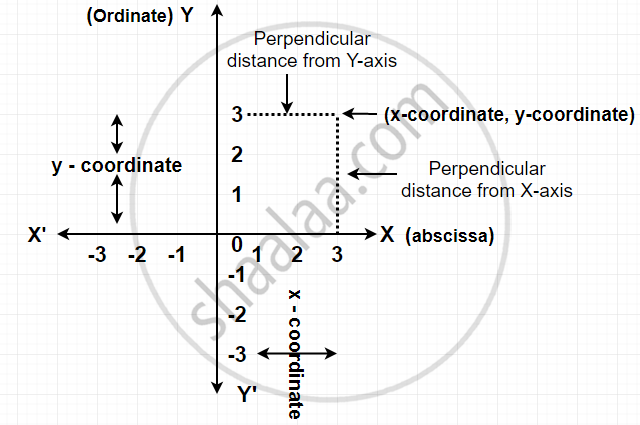## Example

Plot the point (4, 3) on a graph sheet. Is it the same as the point (3, 4)?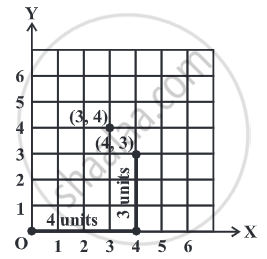The points (3, 4) and (4, 3) are two different points.

## Example

From Fig, choose the letter(s) that indicate the location of the points given below: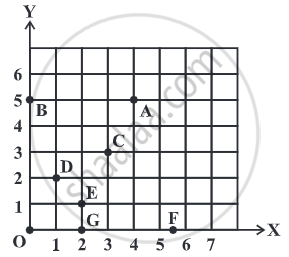(i) (2, 1)
(ii) (0, 5)
(iii) (2, 0)
Also, write
(iv) The coordinates of A.
(v) The coordinates of F.
(i) (2, 1) is the point E.
(ii) (0, 5) is the point B.
(iii) (2, 0) is the point G.
(iv) Point A is (4, 5)
(v) F is (5.5, 0)

## Example

Plot the following points and verify if they lie on a line. If they lie on a line, name it.
(0, 2), (0, 5), (0, 6), (0, 3.5)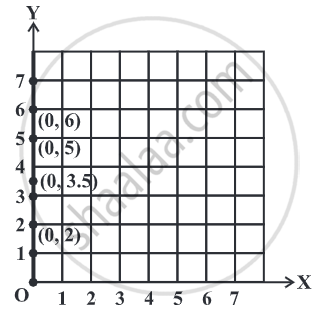These lie on a line. The line is the y-axis.

## Example

Plot the following points and verify if they lie on a line. If they lie on a line, name it.
A (1, 1), B (1, 2), C (1, 3), D (1, 4)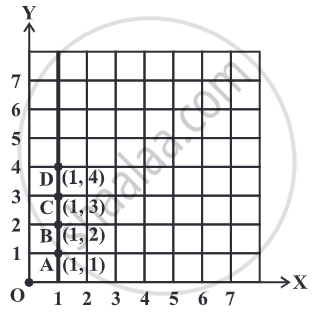These lie on a line. The line is AD.
It is parallel to the y-axis

## Example

Plot the following points and verify if they lie on a line. If they lie on a line, name it.
K (1, 3), L (2, 3), M (3, 3), N (4, 3)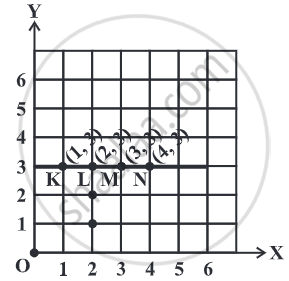These lie on a line. We can name it as KL or KM or MN etc. It is parallel to the x-axis.

## Example

Plot the following points and verify if they lie on a line. If they lie on a line, name it.
W(2, 6), X (3, 5), Y(5, 3), Z (6, 2)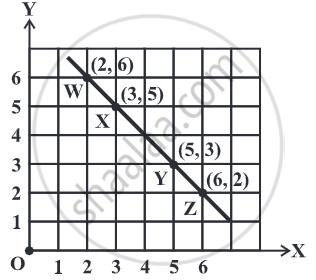These lie on a line. We can name it as XY or WY or YZ etc.
If you would like to contribute notes or other learning material, please submit them using the button below.

### Shaalaa.com

How to Plot points on a graph [00:02:40]
S
0%